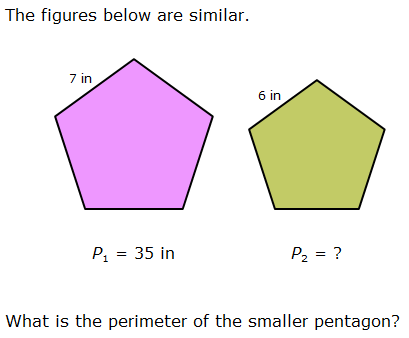# Similarity and congruenceHave a look at  the power point presentation for this unit:

Similarity and congruency

SIMILAR TRIANGLES

Similarity of triangles is a bit like congruence:

• We say that two triangles are congruent if they have the same shape and the same size.
• Two triangles are similar if they have the shape, but they don’t have to have the same size.

When you talk about congruent triangles, you are saying that the corresponding sides’ measures are equal but we’re not making that claim when we say that two triangles are similar!

So how can we tell if two triangles are similar?

It’s pretty simple; if all their pairs of corresponding angles are equal in measure, then the two triangles are similar.

Two triangles are similar if and only its corresponding angles are congruent and corresponding sides are proportional.

Take the diagram below as an example:You can practice Similarities by clicking here.

Interactive excercises to practice with similarity.

You can review the unit with some  similarity problems .

PERIMETERS OF SIMILAR FIGURES:You have to take into account that P1/P2 = k

so, 7/6 = 35/P2

P2 =( 6 x 35) :7 = 30

30 is the perimeter for the smaller pentagon.

AREAS OF SIMILAR FIGURES:That means: A1/A2 = k²

# LES LUTHIERS Y EL TEOREMA DE THALES

Este sitio usa Akismet para reducir el spam. Aprende cómo se procesan los datos de tus comentarios .